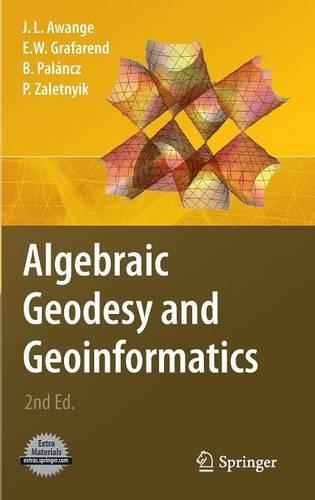•# Algebraic Geodesy and Geoinformatics

(author), (author), (author), (author)
£179.01
Mixed media product 377 Pages / Published: 05/07/2010
• Not available

This product is currently unavailable.

While preparing and teaching 'Introduction to Geodesy I and II' to undergraduate students at Stuttgart University, we noticed a gap which motivated the writing of the present book: Almost every topic that we taught required some skills in algebra, and in particular, computer algebra! From positioning to transformation problems inherent in geodesy and geoinformatics, knowledge of algebra and application of computer algebra software were required. In preparing this book therefore, we have attempted to put together basic concepts of abstract algebra which underpin the techniques for solving algebraic problems. Algebraic computational algorithms useful for solving problems which require exact solutions to nonlinear systems of equations are presented and tested on various problems. Though the present book focuses mainly on the two ?elds, the concepts and techniques presented herein are nonetheless applicable to other ?elds where algebraic computational problems might be encountered. In Engineering for example, network densi?cation and robotics apply resection and intersection techniques which require algebraic solutions. Solution of nonlinear systems of equations is an indispensable task in almost all geosciences such as geodesy, geoinformatics, geophysics (just to mention but a few) as well as robotics. These equations which require exact solutions underpin the operations of ranging, resection, intersection and other techniques that are normally used. Examples of problems that require exact solutions include; * three-dimensional resection problem for determining positions and orientation of sensors, e. g. , camera, theodolites, robots, scanners etc.

Publisher: Springer-Verlag Berlin and Heidelberg GmbH & Co. KG
ISBN: 9783642121234
Number of pages: 377
Weight: 756 g
Dimensions: 235 x 155 x 25 mm
Edition: 2nd ed. 2010